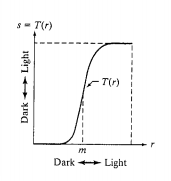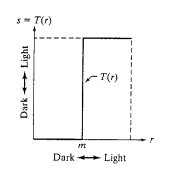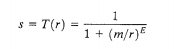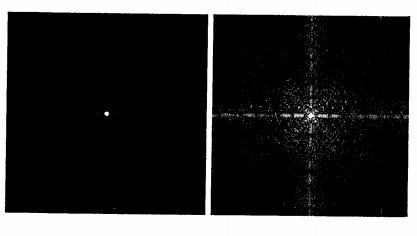#### Introduction

Contrast is created by the difference in luminance, the amount of reflected light, reflected from two adjacent surfaces.

There is also the Weber definition of contrast:
Contrast =(Lmax - Lmin)/Lmax

Lmax = Luminance on the lighter surface
Lmin = Luminance on the darker surface

When the darker surface is black and reflects no light, the ratio is 1. Contrast is usually expressed as percent; the ratio is multiplied by 100. The maximum contrast is thus 100% contrast. It is the difference between the lights and darks in an image. If an image is of low contrast, then the colours will be muted and dull whereas an image of high contrast has bright and vibrant colours.

#### Contrast Stretching

The function shown below is a contrast stretching transformation function because itcompresses the input levels lower than m into a narrow range of dark levels and the values above m into a narrow band of light levels in the output image. The result is an image of higher contrast. In fact, in the limiting case shown below, the output is a binary image.The function is of the formwhere r  represents the intensities of the input image, s the corresponding intensity values in the output image, and E controls the slope of the function. It can be implemented as

eps  is used to prevent overflow if f has any 0 values. Since the limiting value of s is 1, output values are scaled to the range (0,1) in this transformation. In the above figure the shape was obtained with E=20. The following example shows the improvement of contrast using this functionOriginal Image                                                         Result